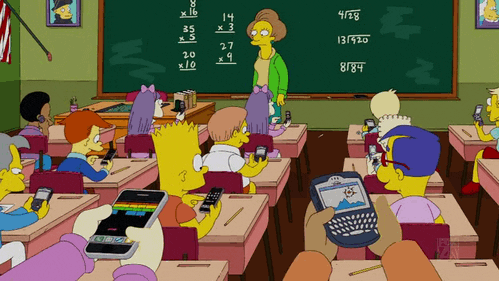Posts Tagged ‘HOmework’Real World Maths: RATES, pop song drumbeats and jogging

September 17, 2020The Rolling Coin Paradox that drove me Crazy!

June 4, 2020The MindYourDecisions blog (Video below) presents the PARADOX beautifully.

Now consider the example of the coin rolling around a coin of the same size. Intuitively we think …’OK. Same circumference, so the coin will rotate once as it rolls around a same-size coin. But this doesn’t happen. It rotates twice.

TWICE!

This observation is SOOOOOOOO counterintuitive we have to know why?

Why?

Mr. MindYourDecisions doesn’t explain why this happens. The answer is interesting. The relevant point in the ROLLING COIN PARADOX is the centre of the rolling coin. The rolling coin rotates about that point. And that centre (the red dot in the gif below) moves through a circle twice the circumference of the stationary coin. It is much easier to understand when you see it. (Below)Make a Cool Pattern with x2 Tables… but it is trickier than you think.

September 6, 2019

A COOL MIDDLE SCHOOL MATH EXERCISE

This idea comes from Burkard and Giuseppe @ the fabulous MATHOLOGER channel. Students can make a pattern called a cardioid that pops up all over math according to Burkard.

Follow these steps. There is a pdf file below the first diagram for printing exercise sheets.

And then watch the MATHOLOGER video for a really interesting explanation.x2 Tables on a Circle pdf file for printing

This circle graph blank could also be used for x3 and x4 tables, which produce totally different yet equally amazing patterns.Halfway there, now it gets tricky. +52 to each point on the circle and keep multiplying by 2.

ie. 27 x 2 = 54, 28 x 2 = 56 and so on.

so 0 = 52, 1 = 53, 2 = 54, 3 = 55, 4 = 56 etcThis shape is called a CARTIOID.Math Joke 1: How to Die from MATH HOMEWORK!

February 25, 2019

NOTE: Homework has never been recorded as the cause of death of a 13 year old.Read longer version of Hugo Does His Homework here.This is why students HATE algebra

January 31, 2019So Mathspig is helping the kid next door with Grade 11 Math.

Here is the revision Q:This is the sort of Q that makes students hate ALGEBRA. (Solution by Mathspig below)

POINTLESS

GRINDING

This equation looks like a chicken ran through some ink and ran across the page.

Why do so many MATH work sheets look like this?

The result:THE ALTERNATIVE

One way to tackle a long and complexmath  problem is to defront the classroom and solve it in groups.ALGEBRA Q from HELL SOLUTION

Here is the Q in orginal form:NOTE: With a proof, you have to prove that one side of the equation

equals the other.MATH JOKE 10: Seriously Funny

January 16, 2019We are good at graphs in maths, even funny graphs, but we often forget the power of story telling. Here’s a story about HOW NOT TO DO your MATHS HOMEWORK*.

*NOTE: Homework has never been recorded as the cause of death of a 13 year old.Read longer version of Hugo Does His Homework here.Sasha a little dog with a BIG, BIG ID Number!

March 28, 2018

This exercise works best if for homework the middle school students count the digits in their own cell phone, passport, bill code or some other number. They don’t have to show the passport or bill. They just have to count the digits.10 Reasons Why Students HATE Maths: BUSTED

December 17, 20171. Maths is BORING!: BUSTED3.  Everyone Hates Maths Anyway: BUSTED…………………………………………………………..

same thing: BUSTED………………………………………………………………………………

5. It’s Too Hard: BUSTED

Come one, a racoon can do it!or You’re Not: BUSTED………………………………………………………………………….

8. I’ll NEVER use this MATHS: BUSTED………………………………………………………………..

Class: BUSTED……………………………………………………………………………………………

10. They’re Always Giving Us New Stuff: BUSTED